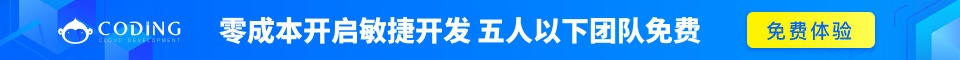# PHP array_splice() 函数完整的 PHP Array 参考手册

### 实例

<?php
\$a1=array("a"=>"red","b"=>"green","c"=>"blue","d"=>"yellow");
\$a2=array("a"=>"purple","b"=>"orange");
array_splice(\$a1,0,2,\$a2);
print_r(\$a1);
?>

## 定义和用法

array_splice() 函数从数组中移除选定的元素，并用新元素取代它。函数也将返回被移除元素的数组。

## 语法

array_splice(array,start,length,array)

array必需。规定数组。
start必需。数值。规定删除元素的开始位置。 0 = 第一个元素。 如果该值设置为正数，则从数组中该值指定的偏移量开始移除。如果该值设置为负数，则从数组末端倒数该值指定的偏移量开始移除。 -2 意味着从数组的倒数第二个元素开始。
length可选。数值。规定被移除的元素个数，也是被返回数组的长度。 如果该值设置为正数，则移除该数量的元素。如果该值设置为负数，则移除从 start 到数组末端倒数 length 为止中间所有的元素。如果该值未设置，则移除从 start 参数设置的位置开始直到数组末端的所有元素。
array可选。规定带有要插入原始数组中元素的数组。如果只有一个元素，则可以设置为字符串，不需要设置为数组。

## 技术细节

返回值： 返回包含被提取元素的数组。 4+

## 实例 1

<?php
\$a1=array("a"=>"red","b"=>"green","c"=>"blue","d"=>"yellow");
\$a2=array("a"=>"purple","b"=>"orange");
print_r(array_splice(\$a1,0,2,\$a2));
?>

## 实例 2

<?php
\$a1=array("0"=>"red","1"=>"green");
\$a2=array("0"=>"purple","1"=>"orange");
array_splice(\$a1,1,0,\$a2);
print_r(\$a1);
?>完整的 PHP Array 参考手册